# Conditional Probability

### Home | Academic Articles

Given events A and B, the probability of A given B is written as P(A | B). This expands into a fraction: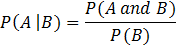In other words, it is the percentage of B that is also in A.

Constructing a 2x2 crosstab

We first introduce the concept of complements.

Example

A = it is raining in Vancouver

Not A = it is not raining in Vancouver

If P(A) = 75%, then P(Not A) = 25%

Complement rule: P(Not A) = 1 – P(A)

It should be noted that some textbook write P(not A) as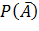.

Example

Suppose 42% of executives read The Wall Street Journal, 8% read The Economist and 2.4% read both publications. Construct a 2x2 crosstab of this.

 WSJ Not WSJ Total EC 2.4 5.6 8 Not EC 39.6 52.4 92 Total 42 58 100

Note that the 42% is in the total cell of the Wall Street Journal and the 8% is in the total cell of The Economist. Since 42% read the Wall Street Journal, this means that 58% do not. Similarly, since 8% read The Economist, this means that 92% do not. These percentages are known as marginals since they are found in the margins of the crosstab.

Note that the 2.4% is in the crosstab of the Wall Street Journal and The Economist since 2.4% of executives read both publications. The remaining percentages are calculated by basic arithmetic. Here is what they represent:

·        The 5.6% is the percentage of executives who read The Economist but not the Wall Street Journal

·        The 39.6% is the percentage of executives who read The Wall Street Journal but not The Economist

·        The 52.4% is the percentage of executives who read neither publication

We can use the crosstab to answer conditional probability questions.

What is the probability an executive reads The Economist given they read The Wall Street Journal?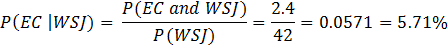What is the probability an executive reads The Wall Street Journal given they read The Economist?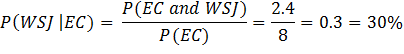Wording issues

Method 1 – use the word “if” in place of the word “given”

Executive example – reword P(WSJ | EC)

What is the probability an executive reads The Wall Street Journal if they read The Economist?

Note that we simply replace the word “given” with the word “if”.

Method 2 – subject/object approach

In the sentence: I like pasta, I is the subject, like is the verb and pasta is the object. In the subject/object approach to conditional probability, we want

P(object | subject).

Executive example – reword P(WSJ | EC)

What percentage of executives who read The Economist also read The Wall Street Journal?

The subject in the above sentence is: executives who read The Economist. (Technically, “who read The Economist” is an adjective phrase modifying executives.) In this question, we are looking at executives who read The Economist and want to determine the percentage of these executives who also read The Wall Street Journal.

Note the difference between these two questions:

What percentage of executives read The Wall Street Journal but not the Economist?

In this question, we are looking at executives as a whole. We want P(WSJ and not EC). From the crosstab, the percentage is 39.6%.

What percentage of executives who read the Wall Street Journal do not read The Economist?

In this question, we are looking at executives who read the Wall Street Journal and want to determine the percentage of these executives who do not read The Economist.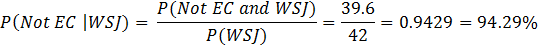It should be noted that P(EC | WSJ) = 5.71% and P(Not EC | WSJ) = 94.29% sum to 100%. The reason is that if an executives read The Wall Street Journal, either they read The Economist or not.